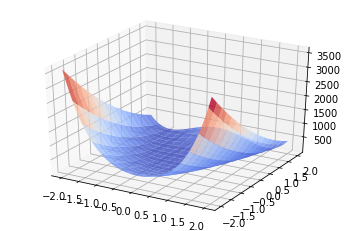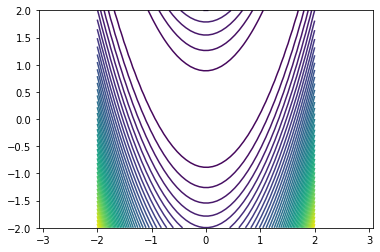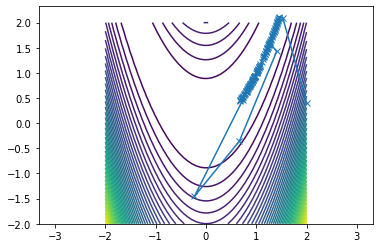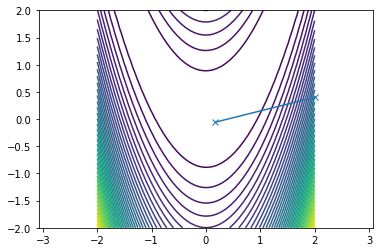# Newton's method in $n$ dimensions¶

In :
import numpy as np
import numpy.linalg as la

import scipy.optimize as sopt

import matplotlib.pyplot as pt
from mpl_toolkits.mplot3d import axes3d


Here are two functions. The first one is an oblong "bowl-shaped" one made of quadratic functions.

In :
def f(x):
return 0.5*x**2 + 2.5*x**2

def df(x):
return np.array([x, 5*x])

def ddf(x):
return np.array([
[1,0],
[0,5]
])


The second one is a challenge problem for optimization algorithms known as Rosenbrock's banana function.

In :
def f(X):
x = X
y = X
val = 100.0 * (y - x**2)**2 + (1.0 - x)**2
return val

def df(X):
x = X
y = X
val1 = 400.0 * (y - x**2) * x - 2 * x
val2 = 200.0 * (y - x**2)
return np.array([val1, val2])

def ddf(X):
x = X
y = X
val11 = 400.0 * (y - x**2) - 800.0 * x**2 - 2
val12 = 400.0
val21 = -400.0 * x
val22 = 200.0
return np.array([[val11, val12], [val21, val22]])


Let's take a look at these functions. First in 3D:

In :
fig = pt.figure()
ax = fig.gca(projection="3d")

xmesh, ymesh = np.mgrid[-2:2:50j,-2:2:50j]
fmesh = f(np.array([xmesh, ymesh]))
ax.plot_surface(xmesh, ymesh, fmesh,
alpha=0.8, cmap=pt.cm.coolwarm, rstride=3, cstride=3)

Out:
<mpl_toolkits.mplot3d.art3d.Poly3DCollection at 0x1201f8050>Then as a "contour plot":

In :
pt.axis("equal")
pt.contour(xmesh, ymesh, fmesh, 50)

Out:
<matplotlib.contour.QuadContourSet at 0x11dd52590>• You may need to add contours to seee more detail.
• The function is not symmetric about the y axis!

### Newton¶

First, initialize:

In :
guesses = [np.array([2, 2./5])]


Then evaluate this cell lots of times:

In :
x = guesses[-1]
s = la.solve(ddf(x), df(x))
next_guess = x - s
print(f(next_guess), next_guess)

guesses.append(next_guess)

0.020912797865820898 [1.14459268 1.30985255]


Here's some plotting code to see what's going on:

In :
pt.axis("equal")
pt.contour(xmesh, ymesh, fmesh, 50)
it_array = np.array(guesses)
pt.plot(it_array.T, it_array.T, "x-")

Out:
[<matplotlib.lines.Line2D at 0x120692b50>]### For comparison: Conjugate Gradients ("CG") -- later in the class¶

Initialize the method:

In :
x0 = np.array([2, 2./5])
#x0 = np.array([2, 1])

iterates = [x0]
directions = [-df(x0)]


Evaluate this cell many times in-place:

In :
# Evaluate this cell many times in-place

x = iterates[-1]
s = directions[-1]

def f1d(alpha):
return f(x + alpha*s)

alpha_opt = sopt.golden(f1d)
next_x = x + alpha_opt*s

g = df(next_x)

beta = np.dot(g, g)/np.dot(last_g, last_g)
directions.append(-g + beta*directions[-1])

print(f(next_x))

iterates.append(next_x)

# plot function and iterates
pt.axis("equal")
pt.contour(xmesh, ymesh, fmesh, 50)
it_array = np.array(iterates)
pt.plot(it_array.T, it_array.T, "x-")

1.4243116772421518

Out:
[<matplotlib.lines.Line2D at 0x1207782d0>]